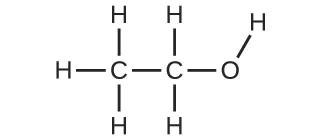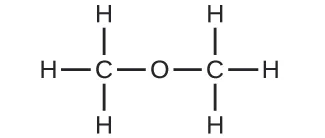Chemistry

# Chapter 2

ChemistryChapter 2

1.

The starting materials consist of one green sphere and two purple spheres. The products consist of two green spheres and two purple spheres. This violates Dalton’s postulate that that atoms are not created during a chemical change, but are merely redistributed.

3.

This statement violates Dalton’s fourth postulate: In a given compound, the numbers of atoms of each type (and thus also the percentage) always have the same ratio.

5.

Dalton originally thought that all atoms of a particular element had identical properties, including mass. Thus, the concept of isotopes, in which an element has different masses, was a violation of the original idea. To account for the existence of isotopes, the second postulate of his atomic theory was modified to state that atoms of the same element must have identical chemical properties.

7.

Both are subatomic particles that reside in an atom’s nucleus. Both have approximately the same mass. Protons are positively charged, whereas neutrons are uncharged.

9.

(a) The Rutherford atom has a small, positively charged nucleus, so most α particles will pass through empty space far from the nucleus and be undeflected. Those α particles that pass near the nucleus will be deflected from their paths due to positive-positive repulsion. The more directly toward the nucleus the α particles are headed, the larger the deflection angle will be. (b) Higher-energy α particles that pass near the nucleus will still undergo deflection, but the faster they travel, the less the expected angle of deflection. (c) If the nucleus is smaller, the positive charge is smaller and the expected deflections are smaller—both in terms of how closely the α particles pass by the nucleus undeflected and the angle of deflection. If the nucleus is larger, the positive charge is larger and the expected deflections are larger—more α particles will be deflected, and the deflection angles will be larger. (d) The paths followed by the α particles match the predictions from (a), (b), and (c).

11.

(a) 133Cs+; (b) 127I; (c) 31P3−; (d) 57Co3+

13.

(a) Carbon-12, 12C; (b) This atom contains six protons and six neutrons. There are six electrons in a neutral 12C atom. The net charge of such a neutral atom is zero, and the mass number is 12. (c) The preceding answers are correct. (d) The atom will be stable since C-12 is a stable isotope of carbon. (e) The preceding answer is correct. Other answers for this exercise are possible if a different element of isotope is chosen.

15.

(a) Lithium-6 contains three protons, three neutrons, and three electrons. The isotope symbol is 6Li or $36Li.36Li.$ (b) 6Li+ or $36Li+36Li+$

17.

(a) Iron, 26 protons, 24 electrons, and 32 neutrons; (b) iodine, 53 protons, 54 electrons, and 74 neutrons

19.

(a) 3 protons, 3 electrons, 4 neutrons; (b) 52 protons, 52 electrons, 73 neutrons; (c) 47 protons, 47 electrons, 62 neutrons; (d) 7 protons, 7 electrons, 8 neutrons; (e) 15 protons, 15 electrons, 16 neutrons

21.

Let us use neon as an example. Since there are three isotopes, there is no way to be sure to accurately predict the abundances to make the total of 20.18 amu average atomic mass. Let us guess that the abundances are 9% Ne-22, 91% Ne-20, and only a trace of Ne-21. The average mass would be 20.18 amu. Checking the nature’s mix of isotopes shows that the abundances are 90.48% Ne-20, 9.25% Ne-22, and 0.27% Ne-21, so our guessed amounts have to be slightly adjusted.

23.

79.904 amu

25.

Turkey source: 26.49% (of 10.0129 amu isotope); US source: 25.37% (of 10.0129 amu isotope)

27.

The symbol for the element oxygen, O, represents both the element and one atom of oxygen. A molecule of oxygen, O2, contains two oxygen atoms; the subscript 2 in the formula must be used to distinguish the diatomic molecule from two single oxygen atoms.

29.

(a) molecular CO2, empirical CO2; (b) molecular C2H2, empirical CH; (c) molecular C2H4, empirical CH2; (d) molecular H2SO4, empirical H2SO4

31.

(a) C4H5N2O; (b) C12H22O11; (c) HO; (d) CH2O; (e) C3H4O3

33.

(a) CH2O; (b) C2H4O

35.

(a) ethanol(b) methoxymethane, more commonly known as dimethyl ether(c) These molecules have the same chemical composition (types and number of atoms) but different chemical structures. They are structural isomers.

37.

(a) metal, inner transition metal; (b) nonmetal, representative element; (c) metal, representative element; (d) nonmetal, representative element; (e) metal, transition metal; (f) metal, inner transition metal; (g) metal, transition metal; (h) nonmetal, representative element; (i) nonmetal, representative element; (j) metal, representative element

39.

(a) He; (b) Be; (c) Li; (d) O

41.

(a) krypton, Kr; (b) calcium, Ca; (c) fluorine, F; (d) tellurium, Te

43.

(a) $1123Na1123Na$; (b) $54129Xe54129Xe$; (c) $3373As3373As$; (d) $88226Ra88226Ra$

45.

Ionic: KCl, MgCl2; Covalent: NCl3, ICl, PCl5, CCl4

47.

(a) covalent; (b) ionic, Ba2+, O2−; (c) ionic, $NH4+,NH4+,$ $CO32−;CO32−;$ (d) ionic, Sr2+, $H2PO4−;H2PO4−;$ (e) covalent; (f) ionic, Na+, O2−

49.

(a) CaS; (b) (NH4)2SO4; (c) AlBr3; (d) Na2HPO4; (e) Mg3 (PO4)2

51.

(a) cesium chloride; (b) barium oxide; (c) potassium sulfide; (d) beryllium chloride; (e) hydrogen bromide; (f) aluminum fluoride

53.

(a) RbBr; (b) MgSe; (c) Na2O; (d) CaCl2; (e) HF; (f) GaP; (g) AlBr3; (h) (NH4)2SO4

55.

(a) ClO2; (b) N2O4; (c) K3P; (d) Ag2S; (e) AlN; (f) SiO2

57.

(a) chromium(III) oxide; (b) iron(II) chloride; (c) chromium(VI) oxide; (d) titanium(IV) chloride; (e) cobalt(II) oxide; (f) molybdenum(IV) sulfide

59.

(a) K3PO4; (b) CuSO4; (c) CaCl2; (d) TiO2; (e) NH4NO3; (f) NaHSO4

61.

(a) manganese(IV) oxide; (b) mercury(I) chloride; (c) iron(III) nitrate; (d) titanium(IV) chloride; (e) copper(II) bromide

Order a print copy

As an Amazon Associate we earn from qualifying purchases.